# Archive | How to with JMP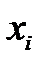## IR Chart with JMP

IR Chart The IR chart (also called individual-moving range chart or I-MR chart) is a popular control chart for continuous data with subgroup size equal to one. The I chart plots an individual observation as a data point. The MR chart plots the absolute value of the difference between two consecutive observations in individual charts [...]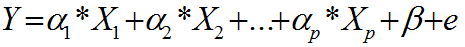## Multiple Linear Regression with JMP

What is Multiple Linear Regression? Multiple linear regression is a statistical technique to model the relationship between one dependent variable and two or more independent variables by fitting the data set into a linear equation. The difference between simple linear regression and multiple linear regression: Simple linear regression only has one predictor Multiple linear regression [...]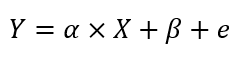## Simple Linear Regression with JMP

What is Simple Linear Regression? Simple linear regression is a statistical technique to fit a straight line through the data points. It models the quantitative relationship between two variables. It is simple because only one predictor variable is involved. It describes how one variable changes according to the change of another variable. Both variables need [...]## One Sample Wilcoxon Test with JMP

What is the One Sample Wilcoxon Test? The one sample Wilcoxon test is a hypothesis test to compare the median of one population with a specified value. Null Hypothesis (H0): η = η0 Alternative Hypothesis (Ha): η ≠ η0 It is an alternative test of one sample t-test when the distribution of the data is non-normal. It is [...]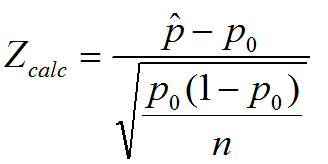## One Sample Proportion with JMP

What is the One Sample Proportion Test? One sample proportion test is a hypothesis test to compare the proportion of one certain outcome (e.g. the number of successes per the number of trials, or the number of defects per the total number of opportunities) occurring in a population following the binomial distribution with a specified [...]## Two Sample t Test with JMP

What is Two Sample t Test? Two sample t test is a hypothesis test to study whether there is a statistically significant difference between the means of two populations. Null Hypothesis (H0): μ1 = μ2 Alternative Hypothesis Ha) : μ1 ≠ μ2 Where: μ1 is the mean of one population and μ2 is the mean of [...]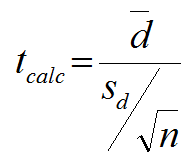## Paired t Test with JMP

What is the Paired t Test? A Third type of a Two Sample t-Test is the Paired t Test.  This test is used when the two populations are dependent of each other, so each data point from one distribution corresponds to a data point in the other distribution. When using a paired t test, the [...]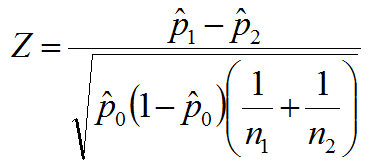## Two Sample Proportion Test with JMP

What is the Two Sample Proportion Test? The two sample proportion test is a hypothesis test to compare the proportions of one certain event occurring in two populations following the binomial distribution. Null Hypothesis(H0): p1 = p2 Alternative Hypothesis(Ha): p1 ≠ p2 Two Sample Proportion Test Assumptions The sample data drawn from the populations of [...]## One Way ANOVA with JMP

What is One Way ANOVA? One way ANOVA is a statistical method to compare means of two or more populations. Null Hypothesis(H0):): μ1 = μ2 ...= μk Alternative Hypothesis(Ha): At least one μi is different, where i is any value from 1 to k It is a generalized form of the two sample t-test since [...]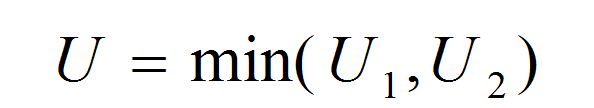## Mann Whitney Testing with JMP

What is the Mann Whitney Test? The Mann Whitney test (also called Mann–Whitney U test or Wilcoxon rank-sum test) is a statistical hypothesis test to compare the medians of two populations that are not normally distributed. In a non-normal distribution, the median is the better representation of the center of the distribution. Null Hypothesis (H0): η1 [...]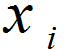## NP Chart with JMP

What is NP Chart? The NP chart is a control chart monitoring the count of defectives. It plots the number of defectives in one subgroup as a data point. The subgroup size of the NP-chart is constant. The underlying distribution of this control chart is binomial distribution. NP Chart Equations NP-chart Data Point: Center Line: [...]## CumSum Chart with JMP

What is a CumSum Chart? The CumSum chart (also called cumulative sum control chart or CUMSUM chart) is a control chart of monitoring the cumulative sum of the subgroup mean deviations from the process target. It detects the shift of the process mean from the process target over time. The underlying distribution of the CumSum [...]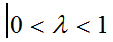## EWMA Chart with JMP

What is EWMA Chart? The EWMA chart (Exponentially-Weighted Moving Average Chart) is a control chart monitoring the exponentially-weighted average of previous and present subgroup means. The more recent data get more weight than older data. It detects the shift of the process mean from the process target over time. The underlying distribution of the EWMA [...]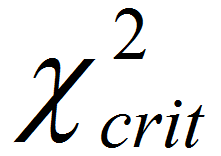## Moods Median Test with JMP

What is Moods Median Test? Mood’s median test is a statistical test to compare the medians of two or more populations. Null Hypothesis (H0): η1 = … = ηk Alternative Hypothesis (Ha): At least one of the medians is different from the others The symbol k is the number of groups of our interest and [...]## Pareto Analysis with JMP

What is the Pareto Principle? The Pareto principle is an observation not a law.  Named after Italian economist Vilfredo Pareto, this principle states that for a variety of situations, 80% of consequences come from 20% of the causes, thus the Pareto principle is also known as the 80/20 rule. The 80/20 rule basically reminds us that [...]